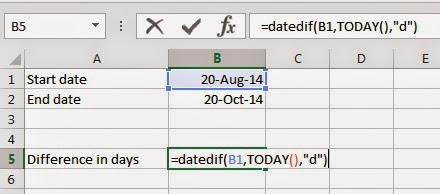## 31 Aug 2014

### Calculate difference between two dates

I recently came up with a hidden function in Excel called DATEDIF. As the name suggests the job of this function is to calculate the difference between two given dates (DATE DIF).
I consider this function as hidden because, for some reason Microsoft has decided not to document this function. And because of this you won’t find this function in the Formula Tab. To verify this, try and type =DATE in any cell. You will see, Excel enlists all the functions that start with the word “date” but it doesn’t shows DATEDIF.

Try to understand the syntax and its usage because Excel won’t provide you any help on this function.
The Syntax of DATEDIF function is as follows:

=DATEDIF(Start_Date, End_Date, Unit)

Here, ‘Start_Date’ is the starting date of the period that you wish to calculate. ‘Start_Date’ can be entered as a string within double quotes (like: “1/9/2012”), or you can also supply the dates as a formula (like: =DATE(2012,1,9)).

‘End_Date’ is the last date of the period that you wish to calculate. Similar to ‘Start_Date’, ‘End_Date’ can also be entered as a string, number or a formula.

‘Unit’ specifies the interval by which you want the difference to be returned, which are

1. As complete years
2. As complete months
3. Number of days
4. Returns the difference between the days in ‘Start_Date’ and ‘End_Date’.

Now, let’s take some examples to use DATEDIF function in excel.

Objective: Let’s, say we want to find the number of days from 20 Aug 2014 till todays date.
So, we will try to apply the DATEDIF formula.

‘Start_Date’: In this case our ‘Start_Date’ will be: 20 Aug 2014.‘End_Date’: End_Date will be today’s date. So, instead of entering the today’s date manually we will use the Today() function.
‘Unit’: As we want to find the number of days between the period. So, the ‘Unit’ will be “d”.

This formula results into: 11 days.Lets explore a few more options using the DATEDIF function.

Comment or mail me if you have any queries.

## Disqus

comments powered by Disqus